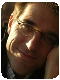DiagramTop achievementsRank 2
Sarper asked on 08 Oct 2012, 08:58 PM
Hi,
I want to make the connectors gradient colored, from source to target. I can't figure out how to do it by setting Stroke style with GradientBrush. Can you help me?

here is what i tried(i know it works for left to right only):
`<``Setter` `Property``=``"Stroke"``>`
`      ``<``Setter.Value``>`
`           ``<``LinearGradientBrush` `StartPoint``=``"0,0"` `EndPoint``=``"1,0"`>
`           ``<``GradientStop` `Color``=``"DimGray"` `Offset``=``"0.0"` `/>`
`           ``<``GradientStop` `Color``=``"Black"` `Offset``=``"1"` `/>`
`       ``</``LinearGradientBrush``>`
`     ``</``Setter.Value``>`
`</``Setter``>`

Thank you,
Sarper

## 5 Answers, 1 is accepted

0Miro Miroslavov
Telerik teamanswered on 10 Oct 2012, 06:53 AM
Hello Sarper,

Do you tried to apply that through style? And how do you apply it? I tried to set the Connection's Stroke as follows and it works as expected.

`<``telerik:RadDiagramShape ``x:Name``=``"s2"``/>`
`<``telerik:RadDiagramShape ``x:Name``=``"s1"``/>`
`<``telerik:RadDiagramConnection ``Source``=``"{Binding ElementName=s1}" ``Target``=``"{Binding ElementName=s2}"``>`
`    ``<``telerik:RadDiagramConnection.Stroke``>`
`        ``<``LinearGradientBrush ``StartPoint``=``"0,0" ``EndPoint``=``"1,0"``>`
`            ``<``GradientStop ``Color``=``"Red" ``Offset``=``"0.0" ``/>`
`            ``<``GradientStop ``Color``=``"Black" ``Offset``=``"1" ``/>`
`        ``</``LinearGradientBrush``>`
`    ``</``telerik:RadDiagramConnection.Stroke``>`
`</``telerik:RadDiagramConnection``>`

It also works for me if I set the Stroke in style setter and then apply that style using the RadDiagram ConnectionStyle Property.

`<``Style ``TargetType``=``"telerik:RadDiagramConnection" ``x:Key``=``"ConnectionStyle"``>`
`    ``<``Setter ``Property``=``"Stroke"``>`
`        ``<``Setter.Value``>`
`            ``<``LinearGradientBrush ``StartPoint``=``"0,0" ``EndPoint``=``"1,0"``>`
`                ``<``GradientStop ``Color``=``"Red" ``Offset``=``"0.0" ``/>`
`                ``<``GradientStop ``Color``=``"Black" ``Offset``=``"1" ``/>`
`            ``</``LinearGradientBrush``>`
`        ``</``Setter.Value``>`
`    ``</``Setter``>`
`</``Style``>`

`<``telerik:RadDiagram ``x:Name``=``"diagram" ``ConnectionStyle``=``"{StaticResource ConnectionStyle}" ``/>`

Hope this helps.

All the best,
Miro Miroslavov
the Telerik team

0Sarper
Top achievementsRank 2
answered on 10 Oct 2012, 07:18 AM
Hi Miro,
The main issue is that style applied only if the link is left to right. But other connections like up-down, down-up, right-left does not work. They works but not source to target, only left to right.

The Start and Endpoint 0,0 1,0 is :

(0,0)________________________________(1,0)

source_________________________target     this works because it's in right direction.

target___________________________source this does not work because style start/end point is 0,0 - 1,0 and the gradient will be target-source direction.

I am using that style:
`<``Style`  `TargetType``=``"telerik:RadDiagramConnection"` `x:Key``=``"ConnectionStyle"``>`
`            ``<``Setter` `Property``=``"ContentTemplate"`  `>`
`                ``<``Setter.Value``>`
`                    ``<``DataTemplate``>`
`                        ``<``TextBlock` `Text``=``"{Binding Name}"``/>`
`                    ``</``DataTemplate``>`
`                ``</``Setter.Value``>`
`            ``</``Setter``>`
`            ``<``Setter` `Property``=``"BezierTension"` `Value``=``"0.5"` `/>`
`            ``<``Setter` `Property``=``"ConnectionType"` `Value``=``"Bezier"` `/>`
`            ``<``Setter` `Property``=``"StrokeThickness"` `Value``=``"2"` `/>`
`            ``<``Setter` `Property``=``"Stroke"``>`
`                ``<``Setter.Value``>`
`                    ``<``LinearGradientBrush` `StartPoint``=``"0,0"` `EndPoint``=``"1,0"``>`
`                        ``<``GradientStop` `Color``=``"DimGray"` `Offset``=``"0.0"` `/>`
`                        ``<``GradientStop` `Color``=``"Black"` `Offset``=``"1"` `/>`
`                    ``</``LinearGradientBrush``>`
`                ``</``Setter.Value``>`
`            ``</``Setter``>`
`        ``</``Style``>`

`<``telerik:RadDiagram` `x:Name``=``"diagram"` `Grid.Row``=``"1"` `SelectionMode``=``"Single"` `ConnectionStyle``=``"{StaticResource ConnectionStyle}"` `Background``=``"Black"` `IsBackgroundSurfaceVisible``=``"False"``/>`

Hope you can understand that issue.
Thank you,
Sarper
0Francois Vanderseypen
Top achievementsRank 2
answered on 10 Oct 2012, 09:11 AM
You need a custom connection and some math to do what you're looking for. The custom implementation below is just an override of the update and considers the different angles (tangents really). Probably there is a more clean solution than the multiple if-then-else but I kept it this way to make the logic clear for you.

`public` `class` `GradientConnection : RadDiagramConnection`
`{`
`    ``private` `readonly` `LinearGradientBrush grad;`

`    ``private` `readonly` `Color startColor;`
`    ``private` `readonly` `Color endColor;`
`    ``public` `GradientConnection()`
`    ``{`
`        ``this``.grad = Application.Current.Resources[``"Grad"``] ``as` `LinearGradientBrush; ``//not that you the start/end are frozen and cannot be reused`
`        ``if` `(``this``.grad == ``null``) ``throw` `new` `Exception(``"Connection gradient template not found."``);`
`        ``this``.startColor = ``this``.grad.GradientStops.Color;`
`        ``this``.endColor = ``this``.grad.GradientStops.Color;`
`        ``var startStop = ``new` `GradientStop(``this``.startColor, 0);`
`        ``var endStop = ``new` `GradientStop(``this``.endColor, 1);`
`        ``this``.grad = ``new` `LinearGradientBrush(``new` `GradientStopCollection(``new``[] { startStop, endStop }));`
`    ``}`

`    ``protected` `override` `void` `Update(``bool` `isManipulating = ``false``)`
`    ``{`
`        ``base``.Update(isManipulating);`
`        ``var b = ``this``.StartPoint;`
`        ``var e = ``this``.EndPoint;`
`        ``var sx = (e.X - b.X);`
`        ``var sy = (e.Y - b.Y);`
`        ``var startPoint = ``new` `Point(0, 0);`
`        ``var endPoint = ``new` `Point(0, 0);`
`        ``if` `(Math.Abs(sx) < Utils.Epsilon) ``//vertical`
`        ``{`
`            ``if` `(Math.Abs(sy) < Utils.Epsilon) ``// as good as zero length`
`            ``{`
`                ``//  doesn't matter as the visual is as good as epsilon-like`
`            ``}`
`            ``else` `if` `(sy > 0) ``//N->S`
`            ``{`
`                ``startPoint = ``new` `Point(0, 0);`
`                ``endPoint = ``new` `Point(0, 1);`
`            ``}`
`            ``else` `//S->N`
`            ``{`
`                ``startPoint = ``new` `Point(0, 1);`
`                ``endPoint = ``new` `Point(0, 0);`
`            ``}`

`        ``}`
`        ``else` `if` `(sx > 0) ``// NE or SE`
`        ``{`
`            ``if` `(Math.Abs(sy) < Utils.Epsilon) ``// horizontal E->W`
`            ``{`
`                ``startPoint = ``new` `Point(1, 0);`
`                ``endPoint = ``new` `Point(0, 0);`
`            ``}`
`            ``else` `if` `(sy > 0) ``//NE->SW`
`            ``{`
`                ``startPoint = ``new` `Point(0, 0);`
`                ``endPoint = ``new` `Point(1, 1);`
`            ``}`
`            ``else` `//SE->NW`
`            ``{`
`                ``startPoint = ``new` `Point(0, 1);`
`                ``endPoint = ``new` `Point(1, 0);`

`            ``}`
`        ``}`
`        ``else` `// NW or SW`
`        ``{`
`            ``if` `(Math.Abs(sy) < Utils.Epsilon) ``// horizontal W->E`
`            ``{`
`                ``startPoint = ``new` `Point(0, 0);`
`                ``endPoint = ``new` `Point(1, 0);`
`            ``}`
`            ``else` `if` `(sy > 0) ``//NW->SE`
`            ``{`
`                ``startPoint = ``new` `Point(1, 0);`
`                ``endPoint = ``new` `Point(0, 1);`
`            ``}`
`            ``else` `//SW->NE`
`            ``{`
`                ``startPoint = ``new` `Point(1, 1);`
`                ``endPoint = ``new` `Point(0, 0);`
`            ``}`
`        ``}`

`        ``this``.grad.StartPoint = startPoint;`
`        ``this``.grad.EndPoint = endPoint;`
`        ``this``.Stroke = ``this``.grad;`
`    ``}`
`}`

The "Grad" is simply a way to allow changing the colors in XAML but you could define a set of custom properties as well of course.To use this custom connection simply use something like:

`public` `partial` `class` `MainWindow : Window`
`    ``{`
`        ``public` `MainWindow()`
`        ``{`
`            ``InitializeComponent();`
`            ``Loaded += OnLoaded;`
`        ``}`

`        ``private` `void` `OnLoaded(``object` `sender, RoutedEventArgs routedEventArgs)`
`        ``{`
`            ``var shape1 = diagram.AddShape(`
`                ``"Source"``, ShapeFactory.GetShapeGeometry(CommonShapeType.CloudShape), ``new` `Point(100, 100));`
`            ``var shape2 = diagram.AddShape(`
`                ``"Target"``, ShapeFactory.GetShapeGeometry(CommonShapeType.RectangleShape), ``new` `Point(300, 300));`
`            ``var con = ``new` `GradientConnection { Source = shape1, Target = shape2, StrokeThickness = 4 };`
`            ``diagram.AddConnection(con);`
`        ``}`

`    ``}`

Hope this helps, Fr.
0Sarper
Top achievementsRank 2
answered on 13 Oct 2012, 06:29 PM
Thank you for the custom connection Francois. It worked like a charm.
But there is some issue with ellipse shape in west and east. I could not understand why.
Maybe it need some calculations but it works in other directions and other shapes.
Thank you,
Sarper
0
AcceptedFrancois Vanderseypen
Top achievementsRank 2
answered on 17 Oct 2012, 01:53 PM
Hi Sarper,

There was indeed a small glitch in the code. I have added a full solution which will produce the attached picture.

Hope this helps, Fr.
Tags
DiagramSarper
Top achievementsRank 2Miro Miroslavov
Telerik teamSarper
Top achievementsRank 2Francois Vanderseypen
Top achievementsRank 2